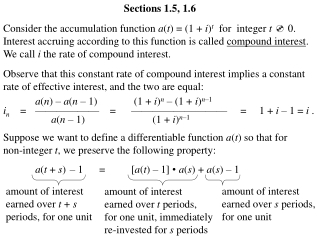DownloadDownload Presentationa ( n ) – a ( n – 1) (1 + i ) n – (1 + i ) n –1

# a ( n ) – a ( n – 1) (1 + i ) n – (1 + i ) n –1

Download Presentation## a ( n ) – a ( n – 1) (1 + i ) n – (1 + i ) n –1

- - - - - - - - - - - - - - - - - - - - - - - - - - - E N D - - - - - - - - - - - - - - - - - - - - - - - - - - -
##### Presentation Transcript

1. Sections 1.5, 1.6 Consider the accumulation function a(t) = (1 + i)t for integer t 0. Interest accruing according to this function is called compound interest. We call i the rate of compound interest. Observe that this constant rate of compound interest implies a constant rate of effective interest, and the two are equal: a(n) –a(n – 1) (1 + i)n– (1 + i)n–1 in = —————— = ————————— = 1 + i – 1 = i . a(n – 1) (1 + i)n–1 Suppose we want to define a differentiable function a(t) so that for non-integer t, we preserve the following property: a(t + s) – 1 = [a(t) – 1] • a(s) + a(s) – 1 amount of interest earned over t + s periods, for one unit amount of interest earned over s periods, for one unit amount of interest earned over t periods, for one unit, immediately re-invested for s periods

2. In other words, we want a(t + s) = a(t) • a(s) . Observe that this property is true for the compound interest accumulation function a(t) = (1 + i)t but not for the simple interest accumulation function a(t) = 1 + it . That is, (1 + i)t + s = (1 + i)t (1 + i)s , and 1 + i(t + s)  (1 + it)(1 + is) .

3. Are compound interest accumulation functions the only ones which preserve the property? For a(t) to be differentiable, we must have a(t + s) – a(t) a(t) •a(s) –a(t) a (t) = lim —————— = lim ——————— s0 ss0 s a(t) (a(s) – 1) a(s) – a(0) = lim ————— = a(t)lim ———— = a(t) •a (0) s0 ss0 s a (t) —— = a (0)  a(t) d — ln[a(t)] = a (0)  dt a (t) = a(t) •a (0)  t t t t d — ln[a(r)] dr = a (0) dr dr ln[a(r)] = r• a (0)  0 0 0 0 t•a (0) ln[a(t)] – ln(1) = t•a (0)  a(t) = e

4. t•a (0) ln[a(t)] – ln(1) = t•a (0)  a(t) = e a (0) a(1) = 1 + i = e a (0) = ln(1 + i)  a(t) = et ln(1+i) We have a(t) = (1 + i)t for all t 0. Consequently, compound interest accumulation functions are the only ones which preserve the property.

5. Observe that (1) (2) With simple interest, the absolute amount of growth is constant, that is, a(t + s) – a(t) does not depend on t. With compound interest, the relative rate of growth is constant, that is, [a(t + s) – a(t)] / a(t) does not depend on t. What is the amount A(0) which must be invested to obtain a balance of 1 at the end of one period? 1 A(0) = —— . 1 + i Since we want 1 = A(1) = A(0) •a(1) = A(0) • (1 + i), then 1 v = —— is called the discount factor. 1 + i What is the amount A(0) which must be invested to obtain a balance of 1 at the end of t periods? Since we want 1 = A(t) = A(0) •a(t), then A(0) = [a(t)]–1. [a(t)]–1 is called the discount function.

6. 1 —— for t 0. 1 + it With a simple interest accumulation function, [a(t)]–1 = With a compound interest accumulation function, [a(t)]–1 = 1 —— = vt for t 0. (1 + i)t a(t) is said to be the accumulated value of 1 at the end of t periods, and [a(t)]–1 is said to be the present value (or discounted value) of 1 to be paid at the end of t periods.

7. Find the present (discounted) value of \$3000 to be paid at the end of 5 years (i.e., the amount which must be invested in order to accumulate \$3000 at the end of 5 years) (a) with a rate of simple interest of 7% per annum. (b) with a rate of compound interest of 7% per annum. (c) with the accumulation function a(t) = 3000 ————— = \$2222.22 1 + (0.07)(5) 3000[a(5)]–1 = 3000 ———— = \$2138.96 (1 + 0.07)5 3000[a(5)]–1 = 3000v5 = t2 1 + — . 25 3000 ———— = \$1500 1 + (52/25) 3000[a(5)]–1 =